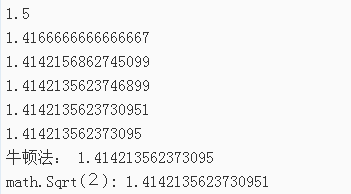﻿ Go指南练习_循环与函数

## 一、练习题描述

`z -= (z*z - x) / (2*z)`

```z := 1.0
z := float64(1)```

（注：如果你对该算法的细节感兴趣，上面的 z² − x 是 z² 到它所要到达的值（即 x）的距离，除以的 2z 为 z² 的导数，我们通过 z² 的变化速度来改变 z 的调整量。这种通用方法叫做牛顿法。它对很多函数，特别是平方根而言非常有效。）

## 二、题目分析

• 定义一个浮点值并对它进行初始化；
• 套用计算公式 z = z - (z*z-x)/(2*z)。

## 三、Go代码

```package main

import (
"fmt"
"math"
)

func Sqrt(x float64) float64 {
z := 1.0// 定义一个初始值并对它初始化
temp := 0.0//  临时变量，作为记录z 上次的值
for {
z = z - (z*z-x)/(2*z)// 计算出最新的z值
fmt.Println(z)
if math.Abs(z-temp) < 0.000000000000001 {
break//  当值停止改变（或改变非常小）的时候退出循环
} else {
temp = z//　赋值最终的结果
}
}
return z
}

func main() {
fmt.Println("牛顿法：", Sqrt(2))
fmt.Println("math.Sqrt(２):", math.Sqrt(2))
}```

运行结果